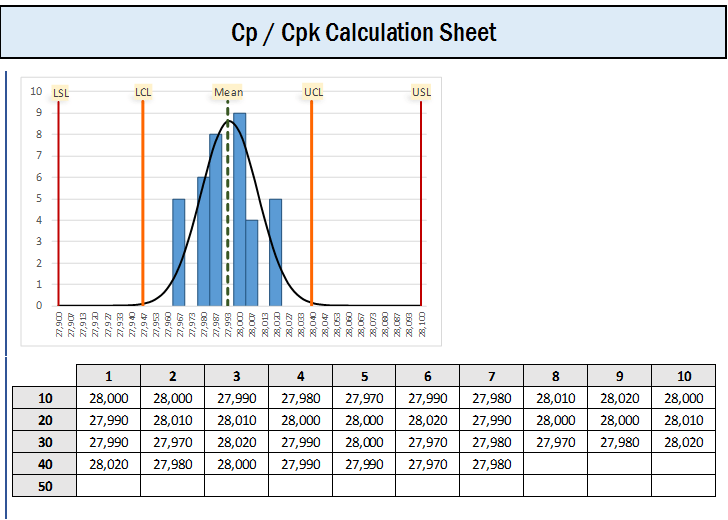To determine how a process is operating, we can calculate:

• Process Capability Index (Cpk).
• Process Capability (Cp).

## Calculating Cpk

Cpk are classified into two; the upper and the lower denoted Cpu and CpL respectively.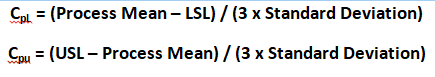We are dividing by 3 because specification limit has an upper bound and a lower bound and since 6 sigmas (i.e. 6 standard deviations) account for nearly all eventualities on a process (assuming normal distribution), hence dividing by 3 enables us to look at only one side of the distribution.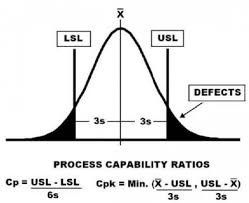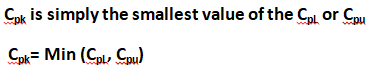## Calculating Cpk using a Z Value

Cpk can be determined by dividing the Z score by 3.Z = x – mean of the population / standard deviation.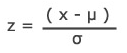## Interpretation of Cpk & Centered Processes

• If a process is perfectly centered, it has a Cp of 1 indicating that the mean was 3 standard deviations (sigma) away from the upper limit and the lower limit.
•  And, a perfectly centered process a process who has a mean exactly in between the 2 specification limits will have a Cpk of 1.

## Deductions from the Values of Cpk

1. Cpk measures how close a process is performing compared to its specification limits and accounting for the inherent process variability.
2. The larger the Cpk value, the less likely the item will be outside the specification limits.
3. When Cpk is negative, the process under study will produce output that is outside the customer specification limits.
4. Cpk is negative when the mean of the process is outside the customer specification limits.
5. At least Cpk = 1.33 [4 sigma] or higher is required to satisfy most customers.
6. Cpk do have upper and lower value reported. If the upper value is 2 and the lower is 1, we say it has been shifted to the left.

## Cpk Values

Cpk = Negative number, process is out of customer’s specification.

Cpk = 0.5, good chance of process meeting customer’s specification.

Cpk = 1, process about meeting customer’s specification.

Cpk = 2, Great! Process meets customer’s specification but can be improved upon.

Cpk =3, Excellent result.

## Calculating Cp

Cp does not account for centering.

Cp = (USL – LSL) / ( 6 x σr )

Cp = (USL – LSL) / (6 x R Bar / d2)

## Cp for Process Mean close to USL

If Process Mean (I.e. central tendency) is closer to the USL, use:

[USL – X (bar) ] / [3 x R Bar / d2], where X (bar) is the Process Mean.

## Cp for Process Mean close to LSL

If Process mean (i.e. central tendency) is closer to the LSL, use:

[x(bar) – LSL ] / [3 x R Bar / d2], where x(bar) is the Process Mean.

## Capability Ratio Cr

The inverse of Cp equals the Capability Ratio (Cr)

Cr = 1/ Cp = ( 6 x σr ) / (USL – LSL)

If Cr < 0.75, the process is capable

If Cr = 0.75 – 1.00, the process is capable with tight control.

If Cr >1, the process is not capable.

## Conclusion on Cp & Cpk

1. If Cp == Cpk, then the process is perfectly centered and, if process is perfectly centered, Cp == Cpk.
2. Cpk can never be larger than Cp, because Cpk accounts for centering (while Cp does not),
3. Both processes are assumed stable.